Next: Marginalising over Areas of Up: Derivation of Similarity Function Previous: Pure Partial Volume Parameters

## Flat Intensity Prior

Initially consider the case of a flat prior on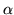. In this case let the range of each individualbe 0 to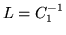such that, then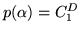. When included,parameters associated with linear intensity gradients have the appropriate columns of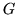scaled so that the range ofis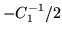to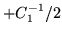, andis still true. Note that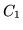is a constant for all, and represents the inverse intensity range.

To start with, take the case where there are no uninteresting, degenerate or partial volume parameters. Note that there may be null parameters. In these conditions, the posterior can be simply calculated using the integrals in appendix A, giving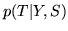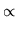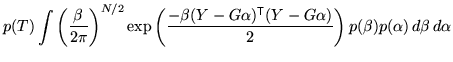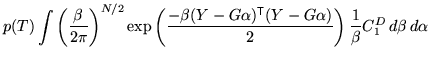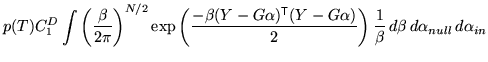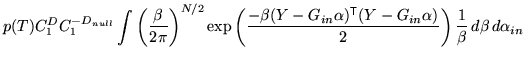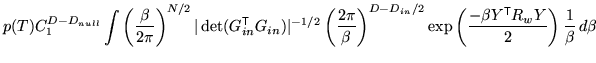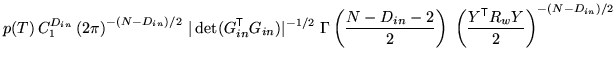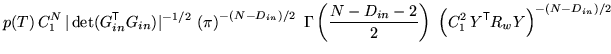(7)

where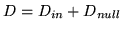,,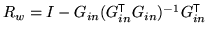, and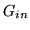is the submatrix formed from thematrix by only including columns associated with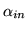parameters. That is,where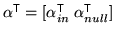.

Note that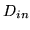andboth depend on the transformation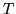. In fact, the dependence onis a form of normalisation for the number of degrees of freedom in the model. Also note that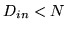in all cases so that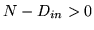and that increasing the normalised residuals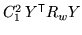causes the posterior probability to decrease, as desired.

The above integrations use the approximation that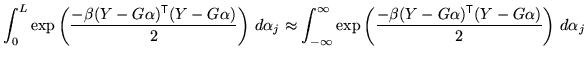which is a good approximation when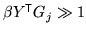and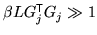. This is true for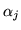parameters where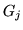(the associated column of) has a norm greater than 1.0 (i.e.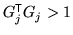), and the true value ofis not within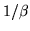of the limits of the range (which can be easily ensured by slightly increasing the prior range). Note that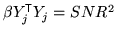, which is the squared Signal to Noise Ratio in voxel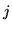and typically much greater than 1.0 in MR images.

When these conditions are not true, the above approximation breaks down and the complimentary error functionterms, as shown in equation 14 in appendix A, must be included. This is true for pure partial volume parameters, and will be treated in section 3.2.2 which will be the last parameters to be integrated over.

SubsectionsNext: Marginalising over Areas of Up: Derivation of Similarity Function Previous: Pure Partial Volume Parameters